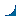>NEW: Info to the keyword feet | >discuss | >create link
on Feb 9th 2003, 13:53:41, Dortessa wrote the following about

# feet

 1 feet = 12 inch = 30.48 cm 1 yard = 3 feet = 36 inch 1 mile = 1760 yard = 5280 feet = 63360 inch = 1609.344 m 1 square mile = 640 acre = 3097600 square yard 1 acre = 4840 square yard = 43560 square feet 1 square feet = 144 square inch = 929.0304 cm² 1 cubic yard = 27 cubic feet = 46656 cubic inch 1 cubic feet = 1728 cubic inch = 28316.846592 cm³
 user rating: +2
Write down what should be considered in connection with »feet«?

Your name:Configuration | Web-Blaster | Statistics | »feet« | FAQ | Home Page0.0015 (0.0006, 0.0002) sek. –– 92196505